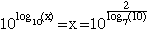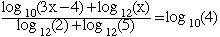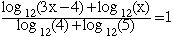Hi..this is one of 9th grade student in Fort Worth TX. well..I am doing EPGY stuffs in my school right now..and.. me and my teacher had problems to solve some advanced logarithmic thing. so I searched some sites to solve these two questions (log base 7 * 10) * (log base 10 * X ) = 2 log base 10 * (3x-4) + log base 12 * X --------------------------------------------------- (log base 12 * 2) + (log base 12 * 5) = log base 10 *4 thanx for caring about this stuff and I hope I can hear from you soon^^ well..thanx again..^^ Hi, I hope I am interpreting these problem correctly. As I understand it the first problem is to solve log7(10) * log10(x) = 2 If so then the solution is log7(10) * log10(x) = 2 so log10(x) = 2/log7(10) Thus,In the second question, to solve for x with the equation below,I think that either one of us is reading something incorrectly or there is a typo in the problem. A better problem is to solve for x with the equationHere the denominator is log12(4) + log12(5) = log12(4*5) = log12(20) and the numerator is log12(3x - 4) + log12(x) = log12((3x - 4)x) = log12(3x2 - 4x) Hence the equation can be written log12(3x2 - 4x) = log12(20) Raising both sides to the twelth power gives 3x2 - 4x = 20 or 3x2 - 4x - 20 = 0 Solve this quadratic for x and then check your answers. Harley Go to Math Central